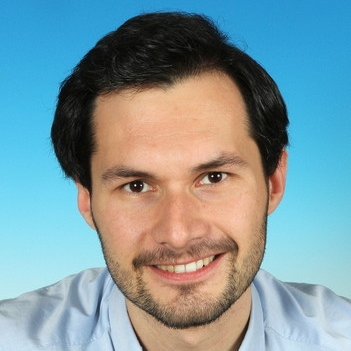# Stretched exponentials and beyond

## PR Abstract

Many mathematical problems begin with a simple question: "How many … are there?" Its innocent and sometimes naive character hides the fact that its answer is often not only difficult but even impossible. Mathematically, this question belongs to enumerative combinatorics, but it appears in many different disciplines, ranging from computer science (e.g., analysis of algorithms) to biology (e.g., phylogenetic trees). Of primary interest are universal phenomena in large random structures. These describe the observation that many combinatorial structures are influenced by only a few global properties and do not depend on concrete details. This project is devoted to one such phenomenon: the occurrence of stretched exponentials in asymptotic counting. But what does this mean?

A sequence of numbers is asymptotically equivalent to another if their common quotient tends towards 1. The idea here is that for a given complicated number sequence that is difficult to compute, a simpler representation is found that reflects its order of magnitude. In this way, approximations can be calculated efficiently and different number sequences can be compared with each other. For example, there are n!=n*(n-1)*...*2*1 many ways to arrange n different playing cards. An asymptotic formula is given here by Stirling's formula, which shows that n! grows superexponentially. Asymptotic formulas can consist of different components that represent, for example, polynomial or exponential growth. Stretched exponentials are a component rarely observed so far, which roughly speaking lies between polynomial and exponential growth. They have the form a^(n^s) for real numbers a and s as well as a natural number n. Recently, some open problems have been solved in which the existence of stretched exponentials was ultimately responsible for their difficulty.

The aim of this project is to develop generic methods to prove and compute such stretched exponentials. Furthermore, we want to classify a general class of two-parameter recurrence relations that have stretched exponentials. We then want to apply this result to open problems in mathematics, biology, physics or chemistry and hopefully find many new examples for the occurrence of stretched exponentials. Specifically, these are problems in automata theory, the compression of data structures, phylogenetics, algebraic group theory, queueing theory and many more. Our method is characterized by its interdisciplinarity and combines the fields of combinatorics, computer algebra, and complex analysis. Our results allow further in-depth analysis of additional parameters such as the typical running time of algorithms.

## Scientific Abstract

This project aims at shedding light on the large-scale behavior of random discrete structures, such as trees and tree-like structures. Such objects appear in numerous scientific fields, ranging from automata theory in computer science to phylogenetic trees in biology. In each case one is interested in understanding the typical shape of such objects, however the precise questions studied can be as diverse as the objects themselves. There are many conjectures about the behavior of these objects, which are in many cases widely open. Often not even the basic counting problem has been solved. In some cases the difficulties arise due to the still mysterious phenomenon of stretched exponentials m^(n^s), where m>0 and 0<\s<1.

### Research questions and objectives

The aim of the project is to gain new insights into the presence of stretched exponentials in asymptotic enumeration. We plan to address the following subjects:

• developing a generic method to prove Theta-results involving stretched exponentials
• determining the multiplicative constant and the D-finite behavior
• analyzing the shape characteristics of directed acyclic graphs and related structures

### Approach and methods

At the core lies the detailed analysis of a class of two-parameter recurrence relations with special weights. This class is motivated by a weighted model of lattice paths that is bijectively connected to the tree-like structures we will analyze using, e.g., the symbolic method and generating functions. The further analysis then requires the usage of computer algebra in order to numerically and heuristically conjecture and prove the asymptotics. Hereby, we need to analyze ordinary differential equations and work with Newton polygons. Furthermore, we will refine the analysis to derive expressions for the involved constants using, e.g., the saddle point method and singularity analysis.

### Level of originality and innovation

The biggest innovation is a new generic method to prove stretched exponentials and analyze the respective counting sequences. So far the saddle point method is the only generic method for proving such a phenomenon. This is the gap that this research project will close: We will develop a new versatile method to prove stretched exponentials and show that this phenomenon is not as rare as could be expected also proving many open conjectures. Our approach enables a detailed analysis of these objects and gives access to their limit shape characteristics.

## Project Contributors#### Michael Wallner (PI)## Publications

1. Enumerative and Distributional Results for d-combining Tree-Child Networks
Yu-Sheng Chang, Michael Fuchs, Hexuan Liu, Guan-Ru Yu, and Michael Wallner; submitted.
2. Walks avoiding a quadrant and the reflection principle
Mireille Bousquet-Mélou and Michael Wallner; submitted.
3. Phase transitions of composition schemes: Mittag-Leffler and mixed Poisson distributions
Cyril Banderier, Markus Kuba, and Michael Wallner; submitted.
4. The binary digits of n+t
Lukas Spiegelhofer and Michael Wallner; to appear in the Annali della Scuola Normale Superiore di Pisa, Classe di Scienze.
5. Enumeration of d-combining Tree-Child Networks
Yu-Sheng Chang, Michael Fuchs, Hexuan Liu, Guan-Ru Yu, and Michael Wallner; in the Proceedings of the 33rd International Conference on Probabilistic, Combinatorial and Asymptotic Methods for the Analysis of Algorithms (AofA 2022).
6. On the critical exponents of generalized ballot sequences in three dimensions and large tandem walks
Michael Wallner; Aequationes mathematicae, 96, 815-826 (2022).
7. Young tableaux with periodic walls: counting with the density method
Cyril Banderier and Michael Wallner; in the Proceedings of the 33rd International Conference on Formal Power Series and Algebraic Combinatorics (FPSAC21).